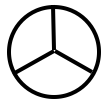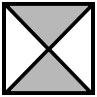Home > Fractions > Misunderstandings > Fractions as a double count

# Fractions as a double count

Tasks such as these are usual in classrooms.

 Colour two thirds of this shape.What fraction of this shape is shaded?Students learn to connect a part-whole area diagram with the written fraction by thinking, "The denominator tells how many parts, and the numerator tells how many are shaded."

While this is a useful basic strategy, it is problematic for several reasons.

• The written fraction is seen as a pair of whole numbers, each recording the count of something different: a 'double count'.
• Many students believe this double count to be the actual definition of a fraction.
• A fraction is not comprehended as a single number, having a value like other numbers.
• The double count cannot be generalised to all situations, such as "Share two pancakes equally among four people."
• Students can lose sight of the whole and interpret a part-whole situation as a ratio, especially when working with fractions of collections.

## Wrong number

The double-count perception of fractions can be detected in student responses to basic tasks.

## Expanding the view

To avoid the limitations of the double-count perception of fractions, students need to expand their view of fractions beyond the basic part-whole model.

## Fraction fiddle

The digital learning object illustrates the relationship between the part-whole model of a fraction and the value of the fraction, by representing its position on a number line.